# Teaching Kids Programming – Compound Interests and Euler’s number (Math Constant E)

Teaching Kids Programming: Videos on Data Structures and Algorithms

Suppose you have deposited \$1 into the bank account, and if the interest rate is 100% (AER = Annual Equivalent Rate).

If the interests are paid out once yearly, together with \$1 (your initial money, also called principal balance), you will have \$1+\$1=\$2.

However, if the interests are paid out twice e.g. every 6 months, you will get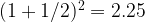$tex_24431e4583a7d5cd153e09f436f04cfb Teaching Kids Programming - Compound Interests and Euler's number (Math Constant E) math python teaching kids programming youtube video$ slightly more: the interests earned at first 6 months contribute to the principal balance in the second 6 months.

If we tend to increase the number of interests paid, we tend to get a larger overall payout (principal and the accumulated interests), however, this does not grow unbounded – in fact, it converges.

The Compound Interests (after a year) can be calculated as: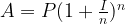$tex_0fc33a12c6b276d05c580cf541b52333 Teaching Kids Programming - Compound Interests and Euler's number (Math Constant E) math python teaching kids programming youtube video$

where: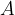$tex_b04e733279486197575c7ed787ae1368 Teaching Kids Programming - Compound Interests and Euler's number (Math Constant E) math python teaching kids programming youtube video$ is the total amount of money you will get, which includes pricipal P and compound interests earned.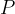$tex_83651ef483f616a73e483203b4efa7fb Teaching Kids Programming - Compound Interests and Euler's number (Math Constant E) math python teaching kids programming youtube video$ is the principal balance aka the initial money deposited.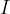$tex_7ce3fd59648a9d37914524180609f7d4 Teaching Kids Programming - Compound Interests and Euler's number (Math Constant E) math python teaching kids programming youtube video$ is the interest rate (AER).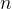$tex_07baee26b11d17fadc32609a4e1b0565 Teaching Kids Programming - Compound Interests and Euler's number (Math Constant E) math python teaching kids programming youtube video$ is the number of interests applied over the period e.g. 1 year.

Translated to Python function:

 ```1 2 ``` ```def A(P, I, n):   return P * (1 + I/n) ** n```
```def A(P, I, n):
return P * (1 + I/n) ** n```

When P=1, I=1 (100%), and n goes to infinity – the A approaches (has a limit of) the Euler’s number – e, the math constant which is roughly,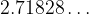$tex_c8594a098622d8ec5f7d5ca53faecb59 Teaching Kids Programming - Compound Interests and Euler's number (Math Constant E) math python teaching kids programming youtube video$ (irrelational number).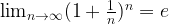$tex_710e6d5c88219799a99ea83064432a66 Teaching Kids Programming - Compound Interests and Euler's number (Math Constant E) math python teaching kids programming youtube video$

GD Star Rating
a WordPress rating system
666 words
Last Post: Teaching Kids Programming - Minimum Genetic Mutation via Iterative Deepening Search Algorithm
Next Post: Teaching Kids Programming - Minimum Sum of Four Digit Number After Splitting Digits

The Permanent URL is: Teaching Kids Programming – Compound Interests and Euler’s number (Math Constant E) (AMP Version)